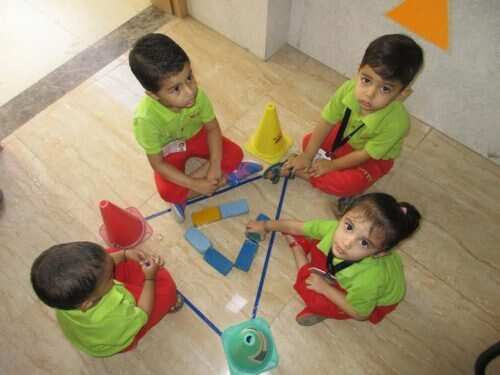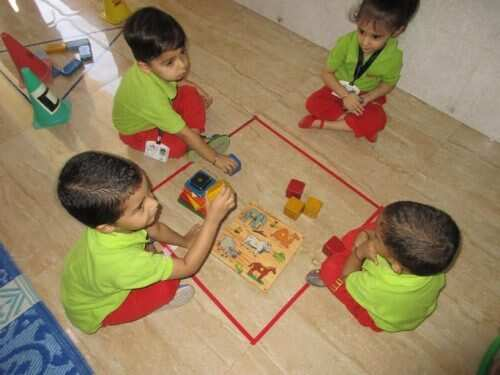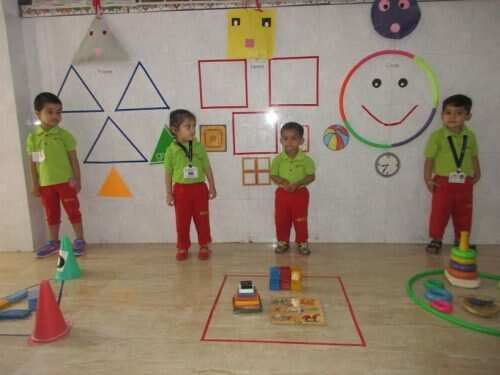# Show and Tell activity organised at Witty

Here is a circle Here is a circle How can you tell? How can you tell? It goes round and round, No end can be found. Here is a triangle Here is a triangle How can you tell? How can you tell? It has 3 sides Here is a square Here is a square How […]Here is a circle

Here is a circle

How can you tell?

How can you tell?

It goes round and round,

No end can be found.

Here is a triangle

Here is a triangle

How can you tell?

How can you tell?

It has 3 sides

Here is a square

Here is a square

How can you tell?

How can you tell?

It has 4 sides, which are same.Children of Playgroup did a recap of all the shapes done so far. They were shown different objects of different shapes and were encouraged to speak about its shape.

They also had a shape walk with their peers. They learnt about the shapes around them.